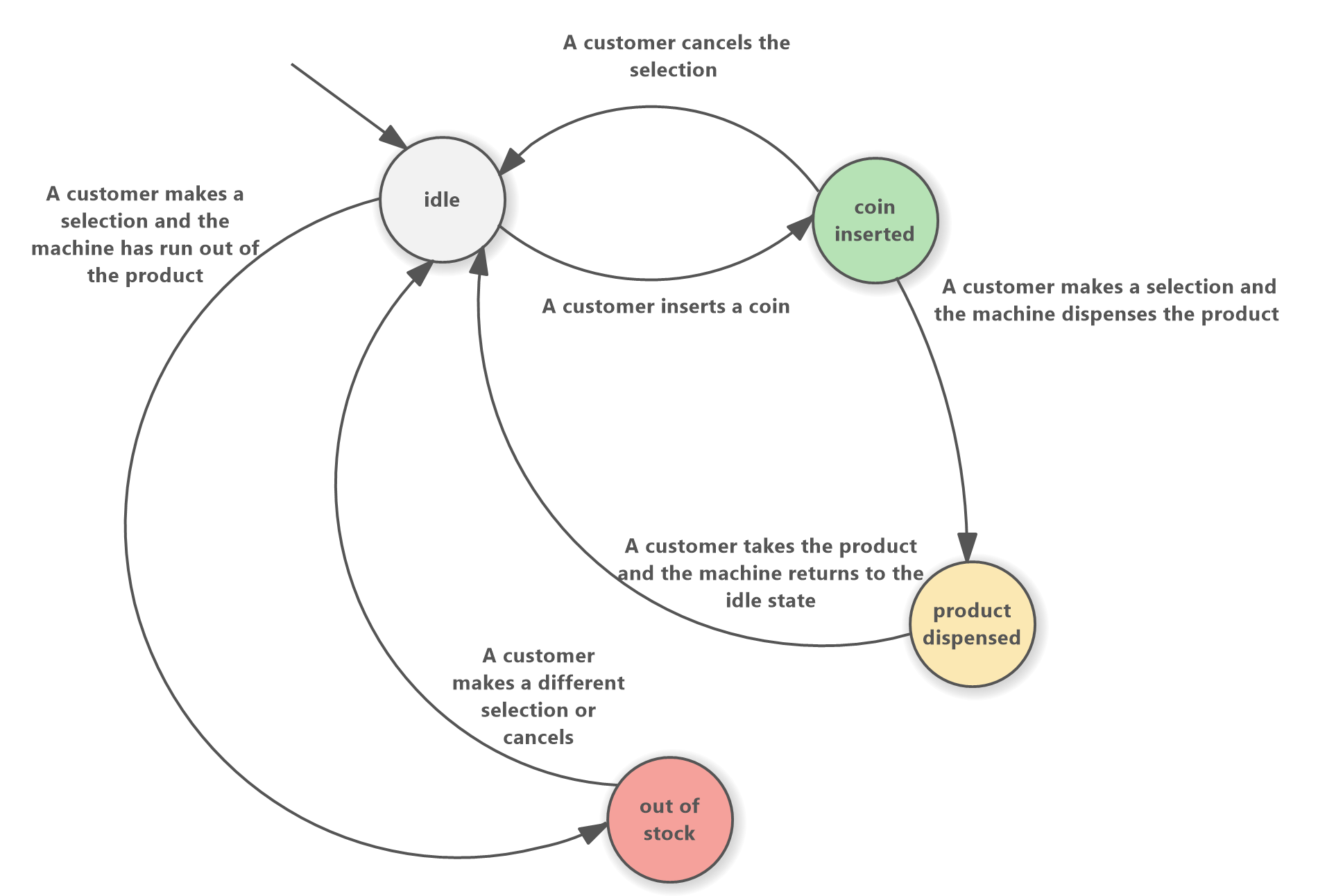# Finite State Machine Diagram

Learn how to create and use Finite State Machine diagrams effectively. The diagram can be used for modeling simple automata as well as complex software systems. Understand the advantages and use cases of FSM diagrams and see a real-life example of how they can be applied.

Finite State Machine diagrams, also known as FSM diagrams or state diagrams, are a type of visual representation used in software engineering, computer science, robotics, and other areas. They are used to model the behavior of a system and are particularly useful for capturing the logic of complex systems. FSM diagrams are often used to model the behavior of software, hardware, and processes with the main focus on the various states of the modeled system.

One of the key features of the FSM diagram is its simplicity and quick learning curve. It consists of State and Transition elements. The State element is represented by a circle and is used to represent a particular state or condition of the system. The Transition element, represented by an arrowed connector, is used to show the transition between two states.

## Create Finite State Machine Diagram

To create a Finite State Machine diagram in Software Ideas Modeler, you can start by selecting the diagram type from the New Diagram menu or dialog. Once the diagram is created, the State and Transition elements will be available in the toolbox.

To add a State element to the diagram, simply click and drag the State element from the toolbox onto the canvas. Then you can give it a name using the displayed text box in the diagram editor.

To add a Transition element, you'll need to decide which two states you want to connect. Then choose the Transition tool from the toolbox and drag from a starting state to an ending state in the diagram editor canvas. This way you can connect the two states. When the transition is inserted, you can enter its name into the text box that appears right after that.

## Finite State Machine Diagram Advantages

Finite State Machine diagrams offer several advantages over other types of diagrams when it comes to modeling the behavior of complex systems. For one, they are easy to understand and can be read by both technical and non-technical stakeholders. They also provide a clear visual representation of the possible states and transitions of a system, making it easy to identify and understand the logic behind the behavior.

In addition, FSM diagrams are also useful for identifying and resolving issues with a system. For example, if you find that a system is behaving unexpectedly, you can use an FSM diagram to trace the flow of the system and identify the state or transition that is causing the problem.

## Finite State Machine Use Cases

Finite State Machine diagrams are particularly useful in a number of different use cases, including:

• Modeling the behavior of hardware systems, such as embedded systems or control systems
• Modeling the behavior of communication protocols
• Modeling the behavior of mechanical systems, such as the behavior of a vending machine or a traffic light controller

Overall, Finite State Machine diagrams are a simple and versatile tool for modeling the behavior of multi-state systems. They provide a clear visual representation of the possible states and transitions of a system and can be used to identify and resolve issues, making them an essential tool for software engineers, hardware engineers, computer scientists, and business analysts alike.

## FSM Diagram Example

This example shows a diagram of a vending machine. The diagram is represented by the different states and transitions that a vending machine can go through during its operation. The states are represented by circles, each labeled with a name such as "idle", "coin inserted", "product dispensed", and "out of stock".An example of finite state machine diagram - Vending Machine

Comment